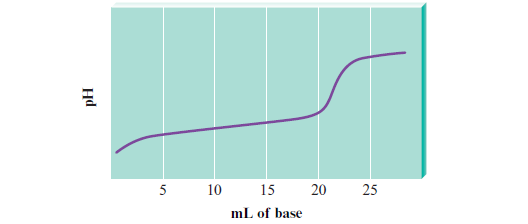# Problem: Consider the titration of a generic weak acid HA with a strong base that gives the following titration curve:On the curve, indicate the points that correspond to the following:a. the stoichiometric (equivalence) point

###### FREE Expert Solution

We are asked to indicate the point that corresponds to the stoichiometric (equivalence) point.

The stoichiometric equivalence point is when the moles acid = moles base

82% (272 ratings)###### Problem Details

Consider the titration of a generic weak acid HA with a strong base that gives the following titration curve:On the curve, indicate the points that correspond to the following:

a. the stoichiometric (equivalence) point